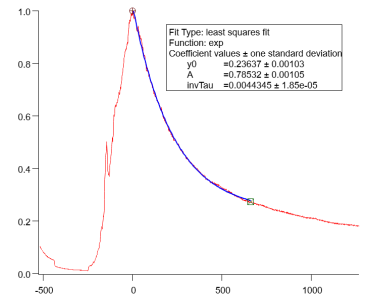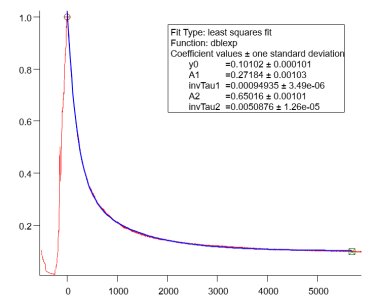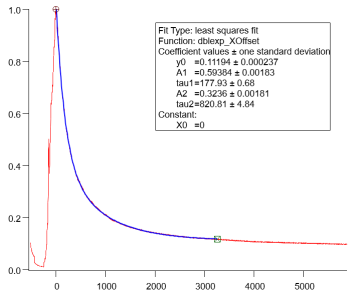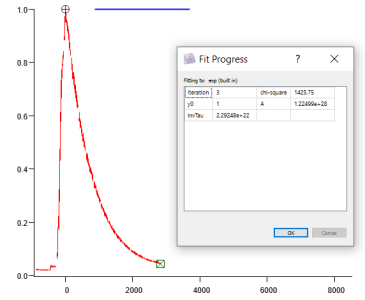# Exp, dbexp and dbexp_XOffset fits, exponential decayHi all,

I’m using the exponential curve fitting which work nicely with my data. I have two questions:

1. I nornalized the data and I was expecting to get y0= 1 since I start the fit at 1(using cursors), but this is not the case (see figures). I went to Analysis-> Curve fitting -> Coefficients and I forced y0= 1 by clicking on hold and make initial guess = 1. In the tab Data options I clicked on "Cursors" (as this is the range fit I'm interested), but the fit I get has no sense, it keeps the fit in 1 (see last figure). How can I do this fitting properly?

2. I want to compare several of these curves. I get nice fits using exp,  dbexp or dbexp_XOffset fit. Since I'm interested in the decay, which fit would make more sense to use?

Thanks!

C.p1) The y0 parameter is the y-offset of the exponential fit, i.e., the value reached at infinity. Note that A is the scaling factor. If I am not wrong, you will get A+y0 = 1 if your fit starts at 1.

2) This depends on your underlying physical model and what you are trying to achieve. Note that dblexp means double exponential. The fit thus uses a combination of two exponential decays, which have, of course, two decay parameters. If this describes your data reality then fine, but I get the feeling that you are just searching for the single exponential decay here.

As a side note: Is there some way to describe the full data set with an appropriate function? In other words, what is the rise? If you have to choose some subset of the data to start fitting in the first place then there is always the arbitrary factor of 'choosing the outcome' left.

A+y0=1 only if the first X value is zero. The amplitude A is projected back to the origin.

The XOffset versions of the exponential functions have a constant X offset Y = y0+ Aexp((x-x0)/tau) in order to accommodate data sets far from the origin without floating-point truncation problems. If you set the x0 constant to the first X value (that is the default) then A+y0 should be close to 1 if your first Y value is 1. Measurement errors might cause a bit of deviation from exactly 1.

When I created the XOffset versions, I took the opportunity to correct an ancient inconvenience- in the XOffset versions, Igor fits the decay constant instead of the inverse decay constant.

You have to be very careful with double exp fits. If the two decay constants are not quite different (in many cases, at least a factor of 3 to 10) then the addition of the second exponential doesn't really change the fit at all, and the two decay constants and amplitudes are only poorly determined. I would say that your double exp fits are on the edge, but since your data has very low noise, it's probably OK.